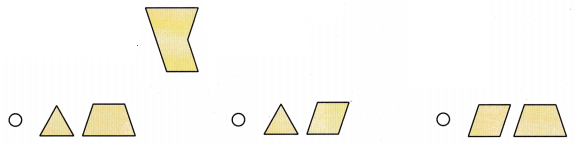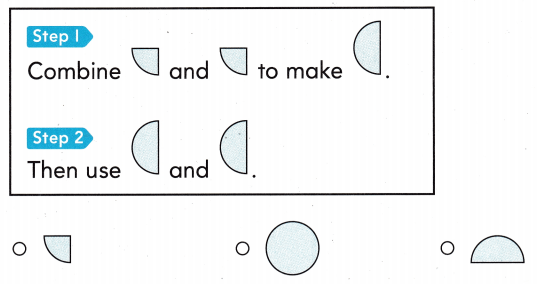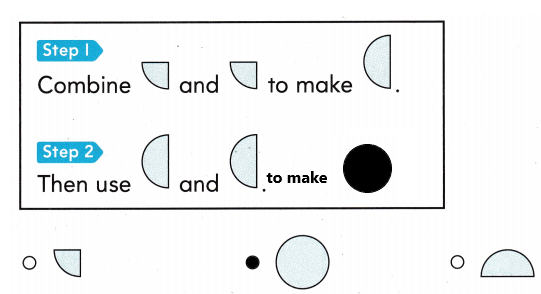Refer to our Texas Go Math Grade 1 Answer Key Pdf to score good marks in the exams. Test yourself by practicing the problems from Texas Go Math Grade 1 Module 14 Assessment Answer Key.

Vocabulary

Match each shape to its name. (pp. 514, 520)

Question 1.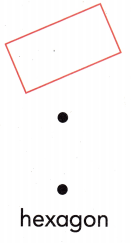• The first and most important property of a regular hexagon is that it has six sides. Hexa means six and therefore a hexagon is supposed to have six sides.

• The next property is in continuation of the first property. As there are six sides, there are also six angles in a hexagon.

• The lengths of each side of a regular hexagon are equal.

• The angles of each side of a regular hexagon are equal.

Question 2.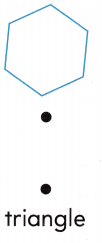Explanation:

• The sum of all the angles of a triangle (of all types) is equal to 180°.
• The sum of the length of the two sides of a triangle is greater than the length of the third side.
• In the same way, the difference between the two sides of a triangle is less than the length of the third side.
• The side opposite the greater angle is the longest side of all the three sides of a triangle.

Question 3.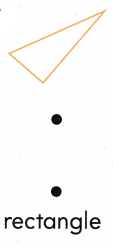Explanation:

• A rectangle is a quadrilateral
• The opposite sides are parallel and equal to each other
• Each interior angle is equal to 90 degrees
• The sum of all the interior angles is equal to 360 degrees
• The diagonals bisect each other
• Both the diagonals have the same length.

Question 4.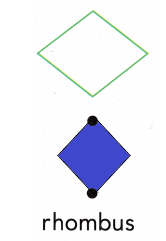Explanation:

• Opposite angles are congruent or equal.
• The opposite sides are equal and parallel.
• Diagonals bisect each other.
• The Sum of any two adjacent or consecutive angles is 180°.

Concepts and Skills

Question 5.
Circle the shapes that have only 3 vertices (corners). (1.6.A)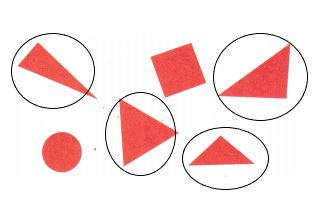• The sum of all the angles of a triangle (of all types) is equal to 180°.
• The sum of the length of the two sides of a triangle is greater than the length of the third side.
• In the same way, the difference between the two sides of a triangle is less than the length of the third side.
• The side opposite the greater angle is the longest side of all the three sides of a triangle.

Use pattern blocks. Draw to show the blocks. Write how many blocks you use. (1.6.C, 1.6.F)

Question 6.
________make a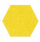.Explanation: The representation has shown in the above diagram. I divided the hexagon with an outline.

Question 7.
_________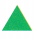make a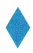.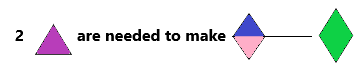Explanation: The representation has shown in the above diagram. I divided the rhombus with an outline.

Question 8.
How many straight sides does a rhombus have? (1.6.D)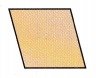(A) 5
(B) 4
(C) 6A rhombus is a diamond-shaped quadrilateral that has all four sides equal. We can see rhombus-shaped figures in our day-to-day lives. Some of the real-life examples of a rhombus are shown in the below-given figure: a diamond, a kite, and an earring, etc.

Question 9.
Which shapes can combine to make this new shape? (1.6.F)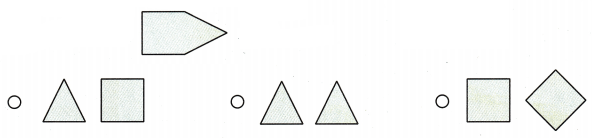Explanation:
The total representation has shown in the above diagram with filled colours.

Question 10.
Which shapes can combine to make this new shape? (1.6.F)